## 7.12Heat transfer in turbulent ﬂow

The initial focus of turbulence modelling is to capture the eﬀect of mixing on momentum diﬀusion since it inﬂuences the overall ﬂow solution. But other properties are also transported by the turbulent eddying motions, in particular heat.

The eﬀects of turbulence on heat transfer can be described using the following equation for internal energy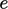, obtained by substituting the material derivative in Eq. (2.57 ) and ignoring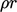: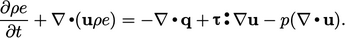(7.44)
In turbulent ﬂow, internal energy can be decomposed into averaged and ﬂuctuating components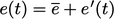, see Eq. (6.11 ). The ensemble average of the terms in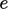introduces a heat ﬂux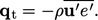(7.45)
This additional heat ﬂux in the energy equation is equivalent to the Reynolds stress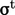, Eq. (6.14 ), in momentum. Boussinesq modelled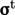by Eq. (6.20 ), using the concept of an eddy viscosity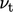corresponding to turbulent mixing, analogous to viscosity due to molecular motion according to Newton’s law Eq. (2.41 ).

Similarly,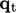can be modelled using a turbulent thermal conductivity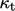due to turbulent mixing, by analogy with Fourier’s law Eq. (2.54 ) for conduction due to molecular interaction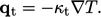(7.46)
The total heat ﬂux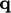in Eq. (7.44 ) is then expressed in terms of the combined turbulent mixing and molecular interaction, using an eﬀective thermal conductivity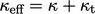, as follows: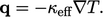(7.47)

### Modelling turbulent heat transfer

Turbulent heat transfer can be incorporated into turbulence models based on eddy-viscosity and Reynolds-averaging, with additional thermal wall functions.

First, the calculation of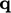by Eq. (7.47) requires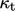from the turbulence model. A common approach to calculate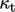is from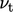based on an estimate of turbulent Prandtl number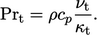(7.48)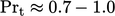provides a good estimate for many ﬂuids, with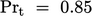often chosen as a default value for CFD calculations. For some more unusual ﬂuids, e.g. liquid metals,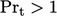.

### Wall heat ﬂux

The calculation of heat transfer through boundary walls is an important aspect of a many CFD simulations. Near walls, the distribution of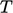tends to mimic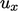.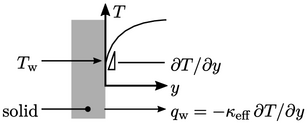Consequently, the challenges of calculating wall heat ﬂux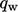are similar to wall shear stress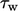. Cells close to the wall must be very thin to resolve the viscous sub-layer in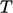(when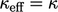).

Otherwise, wall functions can be used to adjust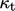to compensate for the under-prediction of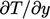as described in Sec. 7.14 .

Notes on CFD: General Principles - 7.12 Heat transfer in turbulent ﬂow